Advertisement.

EnchantedLearning.com is a user-supported site.
As a bonus, site members have access to a banner-ad-free version of the site, with print-friendly pages.
Click here to learn more.

(Already a member? Click here.)

 You might also like: Drawing and Adding Fractions Worksheet Printout #1 Today's featured page: Name Theme Page

 More on Math Drawing and Adding Fractions: Printable Worksheets Fractions Page
 Drawing and Adding Fractions #1:One Half, One Quarter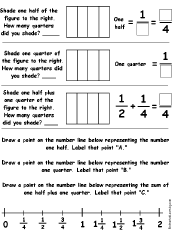Draw and add the fractions one half and one quarter, and plot them on a number line. Or go to the answers. Drawing and Adding Fractions #2:One Half, One Third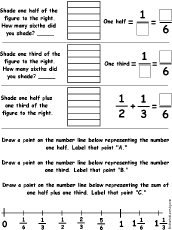Draw and add the fractions one half and one third, and plot them on a number line. Or go to the answers. Drawing and Adding Fractions #3:One Half, One Sixth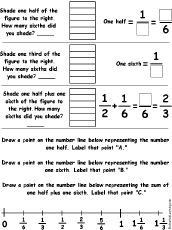Draw and add the fractions one half and one sixth, and plot them on a number line. Or go to the answers. Drawing and Adding Fractions #4:One Half, One Eighth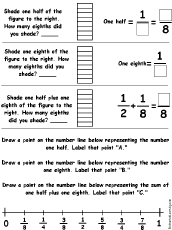Draw and add the fractions one half and one eighth, and plot them on a number line. Or go to the answers. Drawing and Adding Fractions #5:One Half, Three Eighths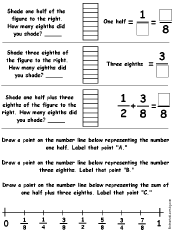Draw and add the fractions one half and three eighths, and plot them on a number line. Or go to the answers. Drawing and Adding Fractions #6:One Quarter, One Eighth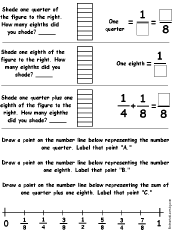Draw and add the fractions one quarter and one eighth, and plot them on a number line. Or go to the answers. Drawing and Adding Fractions #7:One Sixth, One Third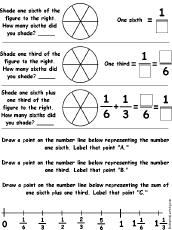Draw and add the fractions one sixth and one third, and plot them on a number line. Or go to the answers. Drawing and Adding Fractions #8:Three Quarters, One Eighth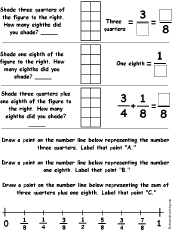Draw and add the fractions three quarters and one eighth, and plot them on a number line. Or go to the answers. Drawing and Adding Fractions #9:Two Thirds, One SixthDraw and add the fractions two thirds and one sixth, and plot them on a number line. Or go to the answers.

## Enchanted Learning Search

 Search the Enchanted Learning website for:

Advertisement.

Advertisement.

Advertisement.

Copyright ©2008-2018 EnchantedLearning.com ------ How to cite a web page